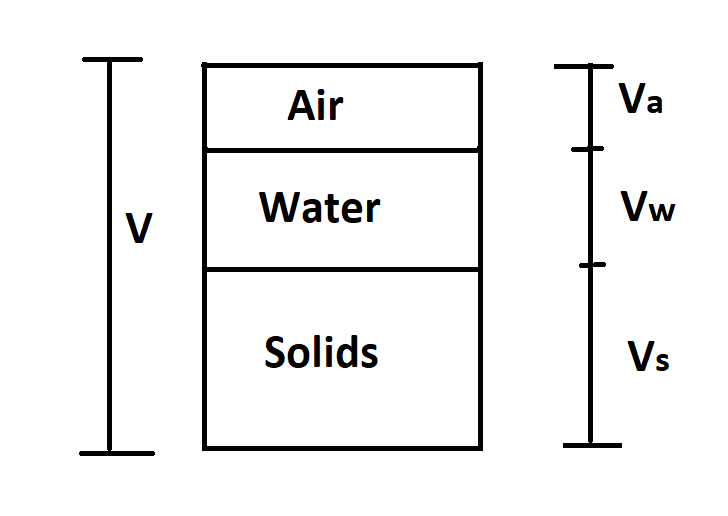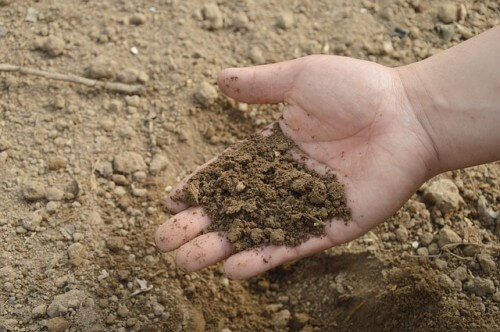Category: soil# Relation Between Dry Unit Weight, Specific Gravity, Percentage of Air Voids, and Water Content

Relation Between Dry Unit Weight, Specific Gravity, Percentage of Air Voids, and Water Content In this article, we shall make the formula or relation between the dry unit weight(), specific gravity(G), percentage of air voids, and water content(w). [Note: Dry unit weight means dry density] Soil three-phase diagram is shown in the above picture. From … Continue reading Relation Between Dry Unit Weight, Specific Gravity, Percentage of Air Voids, and Water Content# Relation Between Void Ratio, Water Content, Degree of Saturation & Specific Gravity

Relation Between Void Ratio, Water Content, Degree of Saturation & Specific Gravity In this article, we will make a formula or equation or relation between void ratio(e), water content(w), degree of saturation() and specific gravity(G). Let, = Weight of soil solid in a given soil mass. = Weight of water. = Volume of soil solid … Continue reading Relation Between Void Ratio, Water Content, Degree of Saturation & Specific Gravity# Relation Between Dry unit Weight, Bulk unit Weight & Water Content

Relation Between Dry unit Weight, Bulk unit Weight & Water Content In this article,we will make the formula/relation between the dry unit weight(), bulk unit weight() and water content(w). [Note: Bulk unit weight means bulk density, and dry unit weight means dry density. So, be careful if the question is like that; derive the relation … Continue reading Relation Between Dry unit Weight, Bulk unit Weight & Water Content

# 5 Empirical Formula for Determination of the Coefficient of Permeability of Soil(K)

The coefficient of permeability of soil(K) can also be computed from several empirical formulae, which are given below: 1. Jaky's Formula. 2. Allen Hazen's Formula. 3. Terzaghi's Formula. 4. Kozney's Formula. 5. Louden's Formula# Capillarity Permeability Test – To Determine the Coefficient of Permeability(K) of Soil

In this article, we are going to discuss about the capillarity permeability test or the horizontal capillarity test, which is another one method used for determining the coefficient of permeability as well as the value of capillarity height of the soil sample.# Dropping Weight Method -To Determine Soil Bearing Capacity

Dropping Weight Method to Determine Soil Bearing Capacity We can determine the bearing capacity of the soil by using the following two methods. Dropping Weight Method Method of Slowly Applying Load In this article, we are discussing the dropping weight method. Dropping Weight Method The dropping weight method is used to determine the approximate value … Continue reading Dropping Weight Method -To Determine Soil Bearing Capacity# How To Verify The Given Bearing Capacity of Any Soil

How To Verify The Given Bearing Capacity of Any Soil We can use the “slowly applying load” method to verify the given bearing capacity of the soil. ⇒ Let's assume the given safe bearing capacity of the soil is 16 t/m².    ⇒ To verify the bearing capacity of this soil, add an initial test load … Continue reading How To Verify The Given Bearing Capacity of Any Soil